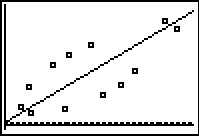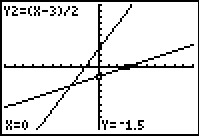••• ##### Device
• TI-83 Plus Family
• TI-84 Plus
• TI-84 Plus Silver Edition
•TI-84 Plus C Silver Edition
•TI-84 Plus CE

# Precalculus: Inverses of Functions

by Texas Instruments#### Overview

Students explore three ways to find the inverse of a function. First, students graph two scatter plots and find the line of reflection. Then, they will graph a line and use the x- and y-intercepts to create the graph of the inverse.

#### Key Steps

•In this activity, students find the inverse of a set of data points by graphing scatter plots. They will then find the line of reflection by finding the midpoint of the first data points and the midpoint of the last data points and connecting them.

•Students will also find the inverse of a line by plotting the points of switched x and y intercept coordinates and connect them with a line. They will then find the inverse algebraically by switching x and y in the equation and solving for y.

There are extra problems at the end of the activity for students to complete either in class or for homework.